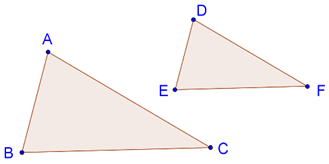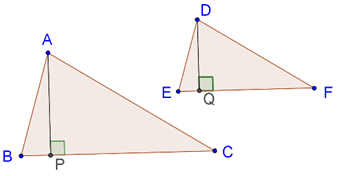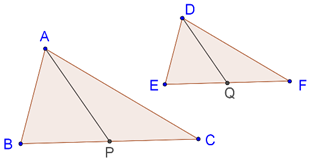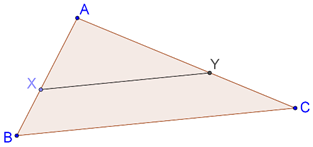# Areas of Similar Triangles

Areas of Similar Triangles
Go back to  'Triangles'

## Introduction to Similarity:

If two triangles are similar it means that:

• All corresponding angle pairs are equal
• All corresponding sides are proportional

However, in order to be sure that two triangles are similar, we do not necessarily need to have information about all sides and all angles.Think: Two congruent triangles have the same area. What about two similar triangles? What is the relation between their areas?

Let us discuss this rigorously.

## Theorem for Areas of Similar Triangles

It states that "The ratio of the areas of two similar triangles is equal to the square of the ratio of any pair of their corresponding sides".

Consider the following figure, which shows two similar triangles, $$\Delta ABC$$ and $$\Delta DEF$$:Theorem for Areas of Similar Triangles tells us that

$\frac{{ar(\Delta ABC)}}{{ar(\Delta DEF)}} = \frac{{A{B^2}}}{{D{E^2}}} = \frac{{B{C^2}}}{{E{F^2}}} = \frac{{A{C^2}}}{{D{F^2}}}$

## Proof of Theorem for Areas of Similar Triangles

Consider two triangles, $$\Delta ABC$$ and $$\Delta DEF$$,

Given: $$\Delta ABC \sim \Delta DEF$$

To prove: $$\frac{{ar(\Delta ABC)}}{{ar(\Delta DEF)}} = {\left( {\frac{{AB}}{{DE}}} \right)^2} = {\left( {\frac{{BC}}{{EF}}} \right)^2} = {\left( {\frac{{AC}}{{DF}}} \right)^2}$$

Construction: Draw the altitudes AP and DQ, as shown below:Proof: Since, $$\angle B = \angle E$$, $$\angle APB = \angle DQE$$

We can note that $$\Delta ABP$$ and $$\Delta DEQ$$ are equi-angular

Hence,

$\Delta ABP \sim \Delta DEQ$

Thus,

$\frac{{AP}}{{DQ}} = \frac{{AB}}{{DE}}$

This further implies that,

$\frac{{AP}}{{DQ}} = \frac{{BC}}{{EF}}....(1)$

Thus,

\begin{align} \frac{{ar(\Delta ABC)}}{{ar(\Delta DEF)}} &= \frac{{\frac{1}{2} \times BC \times AP}}{{\frac{1}{2} \times EF \times DQ}} \hfill \\ &= \left( {\frac{{BC}}{{EF}}} \right) \times \left( {\frac{{AP}}{{DQ}}} \right) \hfill \\ &= \left( {\frac{{BC}}{{EF}}} \right) \times \left( {\frac{{BC}}{{EF}}} \right)....{\text{[from (1)]}} \hfill \\ \Rightarrow \frac{{ar(\Delta ABC)}}{{ar(\Delta DEF)}} &= {\left( {\frac{{BC}}{{EF}}} \right)^2} \hfill \\ \end{align}

Similarly, we can show that

$\boxed{\frac{{ar(\Delta ABC)}}{{ar(\Delta DEF)}} = {{\left( {\frac{{AB}}{{DE}}} \right)}^2} = {{\left( {\frac{{BC}}{{EF}}} \right)}^2} = {{\left( {\frac{{AC}}{{DF}}} \right)}^2}}$

## Solved Examples:

Example 1: Consider two similar triangles, $$\Delta ABC$$ and $$\Delta DEF$$, as shown below:$$AP$$ and $$DQ$$ are medians in the two triangles. Show that

$\frac{{ar\Delta (ABC)}}{{A{P^2}}} = \frac{{ar\Delta (DEF)}}{{D{Q^2}}}$

Solution: Since $$\Delta ABC \sim \Delta DEF$$,

\begin{align} \frac{{AB}}{{DE}} &= \frac{{BC}}{{EF}} \hfill \\ \Rightarrow \frac{{AB}}{{DE}} &= \frac{{\frac{1}{2}BC}}{{\frac{1}{2}EF}} \hfill \\ \Rightarrow \frac{{AB}}{{DE}} &= \frac{{BP}}{{EQ}}....(1) \hfill \\ \end{align}

Also,

$\angle B = \angle E....(2)$

From (1) and (2) and by SAS similarity criterion, We can note that,

\begin{align} \Delta ABP &\sim \Delta DEQ \hfill \\ \Rightarrow \frac{{AB}}{{DE}} &= \frac{{AP}}{{DQ}}....(3) \hfill \\ \end{align}

Now, By Theorem for Areas of Similar Triangles,

\begin{align} \frac{{ar\Delta (ABC)}}{{ar\Delta (DEF)}} &= \frac{{A{B^2}}}{{D{E^2}}} = \frac{{A{P^2}}}{{D{Q^2}}}....[{\text{from (3)}}] \hfill \\ \Rightarrow \frac{{ar\Delta (ABC)}}{{A{P^2}}} &= \frac{{ar\Delta (DEF)}}{{D{Q^2}}} \hfill \\ \end{align}

Example 2: Consider the following figure:It is given that $$XY\parallel AC$$ and divides the triangle into two parts of equal areas. Find the ratio $$AX:XB$$.

Solution: Since $$XY\parallel AC$$, $$\Delta AXY$$ must be similar to $$\Delta ABC$$.

Now, By Theorem for Areas of Similar Triangles,

$\frac{{ar(\Delta ABC)}}{{ar\left( {\Delta AXY} \right)}} = \frac{{A{B^2}}}{{A{X^2}}}....(1)$

Also, $$XY$$ divides the triangle into two parts of equal areas. Thus,

$\frac{{ar(\Delta ABC)}}{{ar\left( {\Delta AXY} \right)}} = 2{\text{ }}....(2)$

From (1) and (2), we have,

\begin{align} \frac{{A{B^2}}}{{A{X^2}}} &= 2 \hfill \\ \Rightarrow \frac{{AB}}{{AX}} &= \sqrt 2 \hfill \\ \Rightarrow \frac{{AB}}{{AX}} - 1 &= \sqrt 2 - 1 \hfill \\ \Rightarrow \frac{{XB}}{{AX}} &= \sqrt 2 - 1 \hfill \\ \Rightarrow \frac{{AX}}{{XB}} &= \frac{1}{{\sqrt 2 - 1}} \hfill \\ \end{align}Challenge: It is given that $$\Delta ABC \sim \Delta XYZ$$. The area of $$\Delta ABC$$ is 45 sq units and the area of $$\Delta XYZ$$ is 80 sq units. $$YZ = 12$$ units.

Find $$BC$$?

⚡Tip: Use Theorem for Areas of Similar Triangles.

Similar Triangles
Similar Triangles
grade 9 | Questions Set 2
Similar Triangles